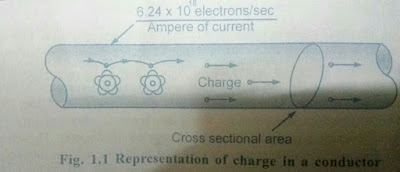# What is Relation Between Electrical Charge And Electrical Current ??

## What is Relation Between  Electrical Charge And Electrical Current ??

About Electrical Charge And Electrical Current

The concept of Electrical Charge in based on atomic theory.

We know that all matter is made up of fundamental building blocks called atoms and that the atoms in turn are composed of different kind of fundamental particles.

The three most important particle are the electron, the proton, and the neutron.Structure Of The Atom

The Electron possesse a negative charge, the proton possesses an equal-magnitude positive charge and the neutron is neutral or has no charge at all.

Recommended Article :

As we rub the rubber comb with woolen cloth, the comb acquires its negative charge because some of the electron on the wool are rubbed off on to the comb.

The cloth then has an insufficient number of electrones to maintain its electrically neutrality and thus behave as a positive charge.

When electron are remove from the substance, that substance becomes positive charged and the substance acquiring electron from the other substance becomes a negatively charged.

The basic unit of charge, called the Coulomb after Charles Coulomb, the first person to make careful quantitative measurments of the force between two charges. The electron has a charge of 1.6021*10^-19 Coulomb.

Here How We Generate Electricity In The
Coal Based Thermal Power Station
Hydro Power Station
Nuclear Power Station

• The phenomenon of transferring charge from one point in a circuit to another point is described by the term, electric current,
• An Electric Current may be define as the time rate of net motion of electric charge across a cross-sectional of the conducting material.

Remember that random motion of free electron in a metal does not constitute a current unless that is a net motion of the charge with time.

FigerRepresentation Of Charge In Conductor

In equation form, the current is
i=dq/dt

If the charge q is in coulombs and time t is measured in second, then the Electrical Current is measured in amperes(after the name of franch physicist Ander Ampere).

A charge in motion constitutes an Electric Current.

1.